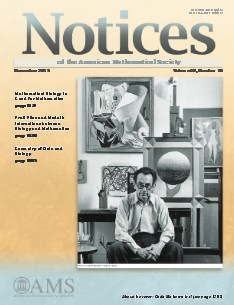## Friday, October 16, 2015

### Mathematical Biology is Good for Mathematics by Michael C. ReedPhoto: Michael C. Reed
Take a closer look at this interesting article on mathematical biology in Notices of the American Mathematical Society  – November 2015 written by Michael C. Reed, professor of mathematics at Duke University.

"About ten years ago I wrote an article, “Why is Mathematical Biology so Hard?” for these Notices intending to explain why the applications of mathematics to biology would be very different than the traditional applications to physics and engineering . A lot has happened since then. Mathematical biology has grown from a small field, containing relatively few mathematicians, to a major branch of applied mathematics. The reasons for this growth, which are implicit in the discussion below, are not the point of this article, nor do I want to encourage mathematicians to switch to mathematical biology.
Rather, I want to make the case that mathematical biology benefits all mathematicians; it is good for the health of mathematics as a whole."Notices

Most of Science is Biology
That statement seems absurd, almost laughable to many mathematicians who are used to thinking that “science” means physics and chemistry, while biology is just classification, necessary perhaps for training doctors, but not really deep, intellectual, or mathematical. We are in the midst of a biological revolution whose roots lie in the 19th and first half of the twentieth century. In the past twenty-five years the pace of this revolution has accelerated and it has created an enormous biological research community.